## Visualizing complex-valued functions with Matplotlib and Mayavi Domain coloring method

The study of complex-valued functions is a difficult chapter of mathematics for most students. Usually textbooks do not show up any figure to visualize in some way such functions.

When studying real functions of one or two variables, an intuitive grasp of some properties one acquires looking at their graphs. Unlike real functions of one or two variables, whose graphs are curves in $\mathbb{R}^2$, respectively surfaces in $\mathbb{R}^3$, the graph of a complex function, $f:\mathbb{C}\to\mathbb{C}$, lies in $\mathbb{R}^4$. Visualization in four dimensions is a difficult task and the method employed depends on the "geometry" of the object to be visualized.

Fortunately, the development of cylindrical color models, such as HSL and HSV, and the possibility to express the values $f(z)$ of a complex function in polar coordinates, $f(z)=|f(z)|\exp(i \arg(f(z))$, led to the design of a fruitful technique of visualization of the values of a complex function through a color-coding method. This method is called domain coloring.

A list of references dedicated to visualization of complex functions, as well as of the software implementations of different methods of visualization can be found in Notices of AMS and on Hans Lundmark's complex analysis pages.

In order to understand the basic idea behind domain coloring we describe the RGB and HSV color model.

### RGB and HSV color model¶

The RGB (Red, Green, Blue) is the basic color space. Its geometrical model is the unit cube. Each color is represented as a triple $(r,g,b)$ of real numbers in $[0,1]$, where $r,g,b$ are quantities of the primary colors (red, green and blue) contained by that color.

In :
from IPython.display import Image
Image(url='http://www.blackice.com/images/RGB_Cube.jpg')

Out: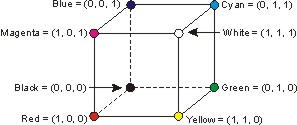Computers treat colors in RGB format. However in applications it is much more appropriate to use the HSV color model, because colors in this space are specified in the same way the humans perceive the color, namely giving the hue, saturation and brightness.

In :
Image(filename='Imags/HSV.png')# a resized image of this one
#http://en.wikipedia.org/wiki/File:HSV_color_solid_cylinder_alpha_lowgamma.png

Out: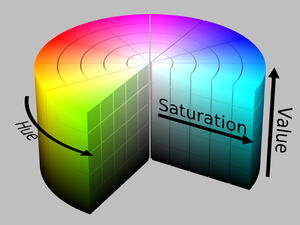The HSV model is represented by a solid cylinder having the radius $1$, and height 1. $H$ is the hue, $S$, saturation, and $V$ represents the brightness.

Sectioning this cylinder with a plane perpendicular to its vertical axis, at the height $V=v$, one gets a color wheel whose complex parameterization is $z=Se^{2\pi i H}$.

As hue $H$ varies from 0 to 1, the corresponding colors vary in this color wheel such that at $2\pi H$, with $H\in\{0, 1/6, 1/3, 1/2, 2/3, 5/6, 1\}$ are located respectively the colors red, yellow, green, cyan, blue, magenta, and red again (see the next image).

Saturation represents the purity of colors. For $S=1$ all colors are pure (do not contain white). As $S$ decreases the colors look more fade.

The brightness increases from zero to 1. At $V=0$, all colours look dark.

The images below illustrate the color wheels of brightness $V=1$, respectively $V=0.5$.

In :
Image(filename='Imags/ColorWheelV1.jpg')

Out: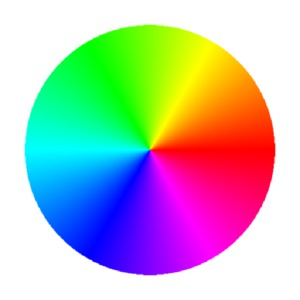In :
Image(filename='Imags/ColorWheelV05.jpg')

Out: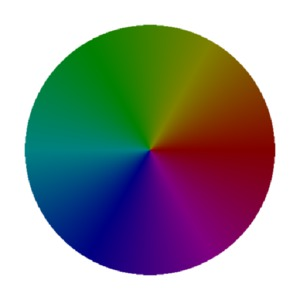In :
%matplotlib inline
import matplotlib.pyplot as plt
import numpy as np
from matplotlib.colors import hsv_to_rgb


Let us get new insights into the HSV model.

In order to see how the color brightness varies in a cylindrical surface of constant saturation, we generate the colors in a rectangle obtained by cutting that cylindrical surface along the generatrice of angular coordinate, $H=0$:

In :
plt.rcParams['figure.figsize'] = 10, 6
sat = [1.0, 0.85, 0.5, 0.25]
svals = ['S=1', 'S=0.85', 'S=0.5', 'S=0.25']
for k, s in zip(range(4), sat):
V, H = np.mgrid[0:1:200j, 0:1:200j]
S = s * np.ones(H.shape)
HSV = np.dstack((H, S, V))
RGB = hsv_to_rgb(HSV)
plt.subplot(2,2,k+1)
plt.imshow(RGB, origin="lower", extent=[0, 1, 0, 1])
plt.xticks([0, 1/6, 1/3, 1/2,  2/3, 5/6, 1],
['$0$', r'$\frac{1}{6}$', r'$\frac{1}{3}$', r'$\frac{1}{2}$', r'$\frac{2}{3}$',
r'$\frac{5}{6}$', '$1$'])
plt.xlabel("$H$")
plt.ylabel("$V$")
plt.title(svals[k])
plt.tight_layout()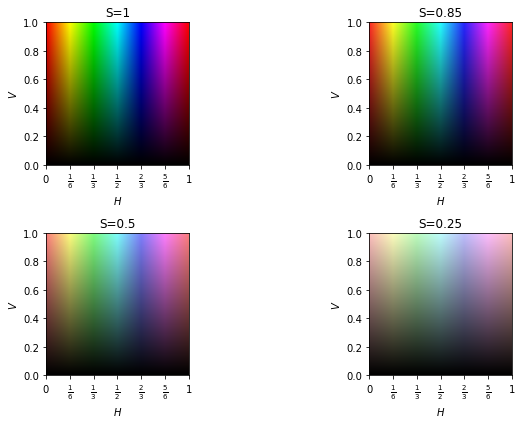These images suggest that the points of a subset $D$ of the complex plane can be encoded by the colors in a rectangle of constant saturation, $S=s_0\in[0,1]$. Namely, to each $z\in D$ one associates the triple $(h, s_0, v)$ in the HSV cylinder, where $h=((\arg(z)/2\pi+1) \:\mbox{modulo}\:1$, and $v=g(|z|)$.

$\arg(z)\in(-\pi, \pi]$ is the principal argument of $z$, and $g:[0,\infty)\to[0,1)$ is a strictly increasing function, chosen such that to be rapidly increasing near zero, in order to avoid too dark colors in the resulting image.

After a few experiments we chose this function:

In :
def g(x):
return (1- 1/(1+x**2))**0.2


Its graph compared with those of other functions of the same type are illustrated here:

In :
plt.rcParams['figure.figsize'] = (6, 4)
x = np.linspace(0, 10, 1000)
y = g(x)
f = lambda z: (1 - 1/(1+z**2))**0.5
h = lambda z: (1.-1/(1+z**2))**0.4
plt.plot(x,y)
plt.plot(x, f(x))
plt.plot(x, h(x))

Out:
[<matplotlib.lines.Line2D at 0x1d2fa5727f0>]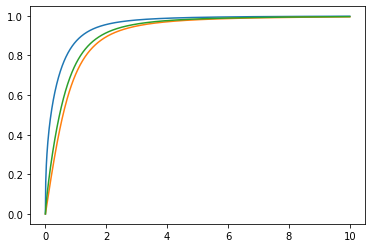The two color models, RGB and HSV, can be converted from one to another. There is no closed form formula for such a conversion. It is only described algorithmically. Here is the pseudocode for the conversion HSV to RGB, and here for RGB to HSV

The Python module colorsys provides functions for these conversions:

(r, g, b) = colorsys.hsv_to_rgb(h, s, v),

respectively

(h, s, v) = colorsys.rgb_to_hsv(r, g, b)

Coordinates of the triples (r, g, b), and (h, s, v), are floating point numbers in $[0,1]$. The hue=h represents the color located at the angular value $2\pi h$ in the color wheel.

For our purposes it is much more useful the function hsv_to_rgb from matplotlib.colors, that converts a numpy array of shape (m, n, 3), and entries in $[0,1]$, defining a HSV colored image, to an array of the same shape, interpreted as a RGB image.

Let us color-encode the points of the rectangular region $D=\{x+iy\in\mathbb{C}\:|\: x\in [-1,4], y\in[-1,3]\}$.

In :
def Hcomplex(z):# computes the hue corresponding to the complex number z
H = np.angle(z) / (2*np.pi) + 1
return np.mod(H, 1)

In :
x = np.arange(-1,4, 0.05)
y = np.arange(-1,3, 0.05)
x, y = np.meshgrid(x,y)
z = x + 1j*y
H = Hcomplex(z)
V = g(np.absolute(z))
S = 0.9*np.ones(H.shape)
HSV = np.dstack((H, S, V))
RGB = hsv_to_rgb(HSV)
plt.imshow(RGB, origin="lower", extent=[-1, 4, -1, 3])

Out:
<matplotlib.image.AxesImage at 0x1d2fa5cb828>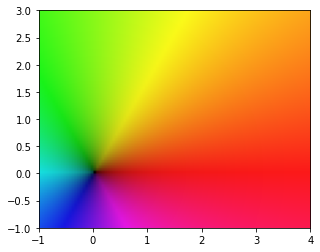One observes that near origin where the modulus is close to 0, the colors are darker.

### Classical domain coloring method¶

Domain Coloring as a method of visualization of complex functions, gained popularity after the publication of the review by F. Farris for the T Needham' book, Visual complex analysis.

In order to visualize a complex function $f$ defined on a rectangular region $[a,b]\times [c,d]\subset\mathbb{C}$, and taking values in the extended complex plane, $\overline{\mathbb{C}}=\mathbb{C}\cup \{\infty\}$, one proceeds as follows:

One defines a narrow grid of this rectangle.

Each node $z$ of the grid will be colored with the HSV color $(h,s_0, v)$, where $h$ and $v$ encode the argument and the modulus of $f(z)$, as described above.

The points $z$ for which $|f(z)|=\infty$ could be colored with white (white is represented by the triple (r,g,b)=(1,1,1), and its conversion to HSV is (0,0,1)).

If we denote by $\mbox{col}:\overline{\mathbb{C}}\to \mbox{HSV}$ the function which encodes the points in the extended complex plane, by a triplet $(h,s, v)$, then the domain coloring is represented by the map $z\mapsto f(z)\mapsto \mbox{col}(f(z))$, i.e. to the point $z$ in the domain one associates the color-code of $f(z)$.

In :
def func_vals(f, re, im,  N): #evaluates the complex function at the nodes of the grid
# re and im are  tuples, re=(a, b) and im=(c, d), defining the rectangular region
# N is the number of discrete points per unit interval

l = re-re
h = im-im
resL = N * l # horizontal resolution
resH = N * h # vertical resolution
x = np.linspace(re, re, int(resL))
y = np.linspace(im, im, int(resH))
x, y = np.meshgrid(x,y)
z = x + 1j*y
return f(z)

In :
def domaincol_c(w, s):#Classical domain coloring
# w is the  array of values f(z)
# s is the constant saturation

H = Hcomplex(w)
S = s * np.ones(H.shape)
modul = np.absolute(w)
V = (1.0-1.0/(1+modul**2))**0.2
# the points mapped to infinity are colored with white; hsv_to_rgb(0, 0, 1)=(1, 1, 1)=white

HSV = np.dstack((H, S, V))
RGB = hsv_to_rgb(HSV)
return RGB

In :
def plot_domain(color_func, f,   re=[-1,1], im= [-1,1], title='',
s=0.9, N=200, daxis=None):
w = func_vals(f, re, im, N)
domc = color_func(w, s)
plt.xlabel("$\Re(z)$")
plt.ylabel("$\Im(z)$")
plt.title(title)
if(daxis):
plt.imshow(domc, origin="lower", extent=[re, re, im, im])

else:
plt.imshow(domc, origin="lower")
plt.axis('off')


In order to read-off some properties of a function $f$, encoded in the generated image, we display alongside the color-code of the complex plane:

In :
plt.rcParams['figure.figsize'] = 8, 5
ab = [-2, 2]
cd = [-2, 2]
plt.subplot(1, 2, 1)
f = lambda z: (z**3 - 1)/z
plot_domain(domaincol_c, f, re=ab, im= cd, title='$f(z)=(z^3-1)/z$', daxis=True)
plt.subplot(1,2,2)
plot_domain(domaincol_c, lambda z:z, re=[-7, 7], im=[-7, 7], title='$z$', daxis=True)
plt.tight_layout()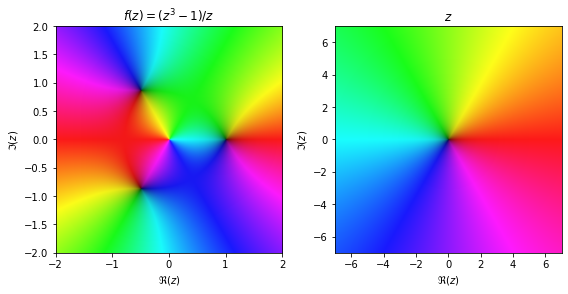Looking at the left image, we observe isochromatic lines. They form the locus of points in the domain that are mapped by $f$ to points having the same argument (i.e points on the rays of the same hue in the right image).

The darker points are mapped by $f$ to points close to the origin.

The function $f$ visualized here has three zeros (the roots of the unity) that are black points, and a pole at $z=0$ (theoretically it is colored white).

This method cannot illustrate however how varies the modulus $|f(z)|$, when it is sufficiently greater than 0. Such an information can be gained from the so called analytic landscape, i.e a surface which is the graph of the modulus $|f(z)|$.

If $|f(z)|$ varies on a too wide range, then one visualizes the logarithmic analytic landcape, that is the graph of the real function $\log(|f(z)|)$.

In the following we give the code for generation of the colored analytic landscape. The surface is colored according to the argument of $f$, using a HSV colormap, where $H$ is defined as for the domain coloring method, and $S$ and $V$ are set to be constant.

This method for coloring an analytic landscape appears to have been proposed by Bernd Thaller and exploited in his book Visual Quantum Mechanics, Springer, 2000.

We are using mayavi.mlab to generate a colored analytic landscape.

In order to run the code below, you must have wxPython installed. Here is the download page.

Otherwise, skip running it.

NOTE! The code below for mayavi plots is unchanged from the first version (2014) of this notebook because mayavi is no more installed on my system. Hence it is possible to be deprecated

In [ ]:
%gui wx

In [ ]:
from mayavi import mlab

In :
def HSVcolormap(): #defines colormap for analytic landscape
argum = np.linspace(-np.pi, np.pi, 256)

H = argum / (2*np.pi) + 1
H = np.mod(H,1)
S = np.ones(H.shape)
V = np.ones(H.shape)

HSV = np.dstack((H,S,V))
RGB = hsv_to_rgb(HSV)

colormap = 255 * np.squeeze(RGB)
return colormap

In [ ]:
def alandscape(rez, imz, argfz, modfz): #draws the (log)analytic landscape using the HSVcolormap

colormap = HSVcolormap()
mesh = mlab.mesh(rez, imz, modfz, scalars=argfz, vmin=-np.pi, vmax=np.pi)

LUT = mesh.module_manager.scalar_lut_manager.lut.table.to_array()
for k in range(3):
LUT[:, k] = colormap[:,k]

mesh.module_manager.scalar_lut_manager.lut.table = LUT
mlab.draw()
return mesh

In :
def evalfun(f, re=[-2,2], im=[-2,2], N=50):# evaluates the log(|f|) at the points of a greed
nrx = N * (re-re)
nry = N * (im-im)
x = np.linspace(re, re, int(nrx))
y = np.linspace(im, im, int(nry))

x, y = np.meshgrid(x, y)
z = x + 1j*y
w = f(z)
w[np.isinf(w)] = np.nan
#m = np.absolute(w) # depending on the function f we can use one of these two versions for computing the module m
m = np.log(np.absolute(w))
return x, y, np.angle(w), m


At this point we are ready to generate the logarithmic analytic landscape of a complex-valued function.

After clicking run in the next cell, one must wait until the mayavi scene is displayed. Then, hovering the mouse over it, the surface can be seen from different points of view. The generated surface is not embedded inline (this was the case in 2014)

In [ ]:
mlab.figure(1, bgcolor=(0.95,0.95,0.95))
x,y, theta, m=evalfun(lambda z: (z**3-1)/z)
alandscape(x, y, theta, m)
mlab.savefig('loglandsc1.jpg' )


Wait a few seconds!!!! The saved image is now displayed:

In :
Image(filename='Imags/loglandsc1.jpg')

Out: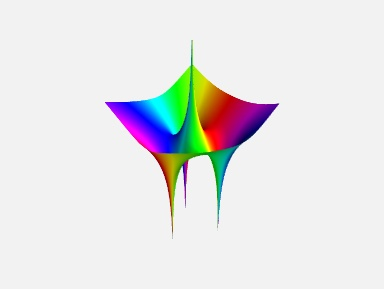The same surface seen from the point of view set by

mlab.view(azimuth=120, elevation=40, distance=15):

In :
Image('Imags/loglandsc2.jpg')

Out: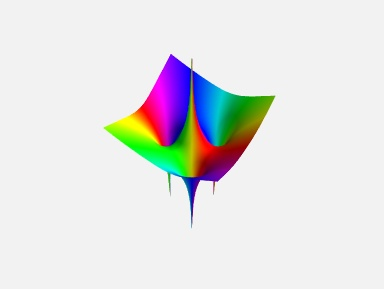The log-analytic landscape of the tangent function:

In :
Image(filename='Imags/logmodultan.jpg')

Out: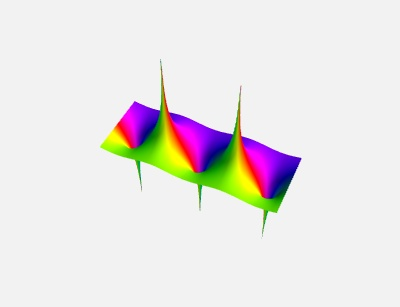LE: In this notebook https://nbviewer.jupyter.org/github/empet/Math/blob/master/Analytic-landscape.ipynb is illustrated how an analytic landscape can be generated via Plotly.

### Domain Coloring with contours lines of constant modulus¶

In order to point out how the modulus of a complex function changes over domain, Lundmark proposed to add a new feature to the classical domain coloring.

For the color function with values in the HSV cylinder, this amounts to define the brightness at a point $z$ as the fractional part of $\log_2(|f(z)|)$, $v=\{\log_2(|f(z)|\}$, where $\{x\}=x-[x]$ ($[x]$ is the integer part of $x$).

Thus when the modulus crosses a value equal to a power of $2$, $|f(z)|=2^k$, $k$ integer, the brightness has a discontinuity and gets dark. Around zeros and poles there are rings delimited by dark lines of constant modulus. Within a ring the brightness changes according to the direction of growth of the modulus.

In :
X = np.linspace(0, 8, 800)
Y = X - np.floor(X)
plt.rcParams['figure.figsize'] = 5, 3
plt.title('The graph of fract(x)')
plt.plot(X,Y,'r')

Out:
[<matplotlib.lines.Line2D at 0x1d2f8ef1cc0>]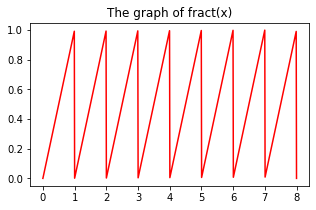The color function implementing this method is defined here:

In :
def domaincol_m(w,  s): #domain coloring with modulus track

# w the array of values
#s is the constant Saturation

H = Hcomplex(w)
modulus = np.absolute(w)
c = np.log(2)
logm = np.log(modulus)/c#log base 2
logm = np.nan_to_num(logm)

V = logm - np.floor(logm)
S = s*np.ones(H.shape)

HSV = np.dstack((H, S, V**0.2)) # V**0.2>V for V in[0,1];this choice  avoids too dark colors
RGB = hsv_to_rgb(HSV)
return RGB


Let us illustrate the domains of a few functions colored by this method:

In :
plt.rcParams['figure.figsize'] = 8, 5

In :
ab = (-2,2)
cd = (-2,2)
plt.subplot(1,2,1)
plot_domain(domaincol_m, lambda z:z,   re=ab, im=cd, title='$f(z)=z$',  daxis=True)
plt.subplot(1,2,2)
f = lambda z: (z**3-1)/z
plot_domain(domaincol_m, f,   re=ab, im=cd, title='$f(z)=(z^3-1)/z$',  daxis=True)
plt.tight_layout()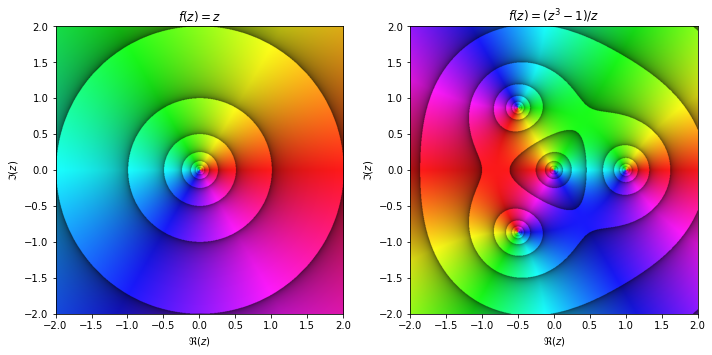In :
plt.rcParams['figure.figsize'] = 10, 6
plt.subplot(1, 2, 1)
ab = (-2, 2)
cd = (-2, 2)
f = lambda z: z**5 + z
plot_domain(domaincol_m, f, re=ab, im=cd, title='$f(z)=(z^5+z)$', daxis=True)
plt.subplot(1, 2, 2)
ab = (-2.5, 2)
cd = (-2.5, 2)
g = lambda z: (z - 0.5 - 0.5*1j) / z**2
plot_domain(domaincol_m, g,   re=ab, im=cd, title='$g(z)=(z-0.5(1+i))/z^2$', daxis=True)
plt.tight_layout()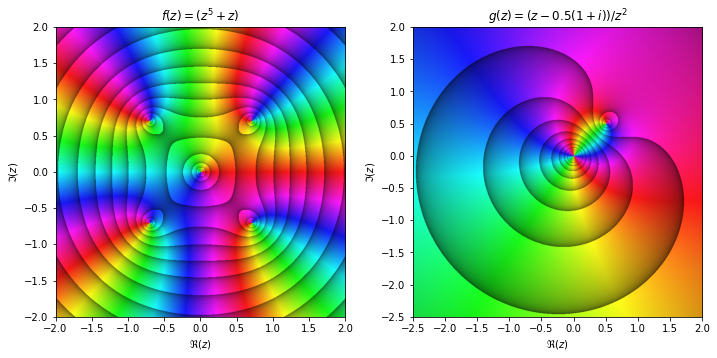In :
plt.rcParams['figure.figsize'] = 12, 6

In :
ab = (-np.pi, np.pi)
cd = (-2, 2)

plt.subplot(1, 2, 1)
plot_domain(domaincol_m, np.sin,   re=ab, im=cd, title='$f(z)=\sin z$', daxis=True)
plt.subplot(1, 2, 2)
plot_domain(domaincol_m, np.tan,   re=ab, im=cd, title='$g(z)=tan(z)$', daxis=True)
plt.tight_layout(1)